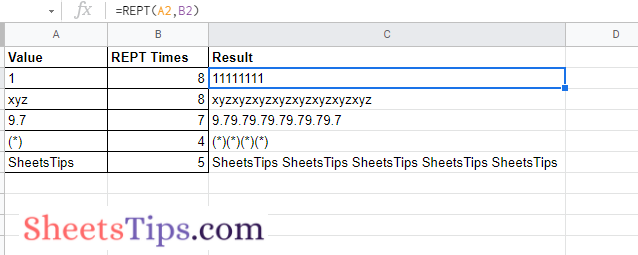# REPT Function In Google Sheets – How To Repeat Rows in Google Sheets?

Google Sheets REPT function will return the text specified times repeatedly. For example, if you want to repeat the term Sheets Tips, thrice, then we can simply use the REPT function to get this done. On this page, let us understand how to use the REPT function in Google Spreadsheet using the Google Sheet Tips provided on this page. Read further to find more.

## REPT Function Syntax in Google Sheets

To use the repeat function in Google Spreadsheet, we will have to use the syntax. The syntax of the REPT function is as follows:

`=REPT(text_to_repeat, number_of_repetitions)`

• text_to_repeat: The letter or string to be repeated is text to repeat.
• number_of_repetitions: Indicates how many times text to repeat should appear in the returned response.

The number of repetitions cannot exceed the 32,000-character maximum of a cell. REPT will return a #VALUE! error if the number of repetitions is larger than 32,000 characters.

### How to Use REPT Function in Google Sheets?

Let us say, we want the term Sheets Tips to be repeated in Google Sheets thrice in a cell. We can get this done in Google Sheets.

Follow the steps as outlined below to use the REPT function in Google Spreadsheet.

• 1st Step: Open the Google Spreadsheet on your device.
• 2nd Step: Now on the homepage, move to the cell where you want the term to get repeated.
• 3rd Step: Enter the formula =REPT(“Sheets Tips “, 3).
• 4th Step: Press the “Return” key and you will see the results as shown below.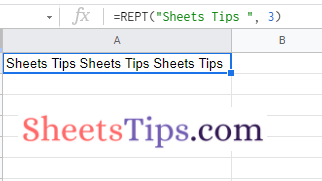If you observe, I have provided extra space after the term “Sheets Tips”. If I remove the space and apply the formula, then the REPT function will print the term without providing any spaces.

### Using REPT Function to Repeat Numbers in Google Sheets

Google Sheets not only repeats the characters but also helps to repeat the numbers. To repeat the numbers in Google Spreadsheet, follow the steps outlined below:

• 1st Step: Launch the Google Spreadsheet on your system.
• 2nd Step: In the Sheet, head to the cell range where you want to apply the REPT function to a number.
• 3rd Step: Now enter the formula =REPT(“4”, 8). Here I am using the number 4 to repeat 8 times using the REPT function.
• 4th Step: Press the “Enter” button and you will see the results.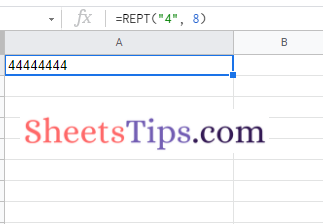### Using REPT Function to Repeat Images in Google Sheets

Google Sheets also allows users to repeat images using the REPT function. The steps to get this done in Google Spreadsheet are as follows:

• 1st Step: Open the Google Spreadsheet.
• 2nd Step: Copy the IMAGE URL which you would like to repeat. Here I am using the Google Logo. So my formula will be “=ArrayFormula(IMAGE(SPLIT(REPT(“https://www.google.com/favicon.ico”&”♕”,5),”♕”)))”.
• 3rd Step: Press the “Return” key and you will see the results.

Note: The Queen symbol “♕” is added at the end of the repeated value to serve as a unique value for the SPLIT function when it divides the string.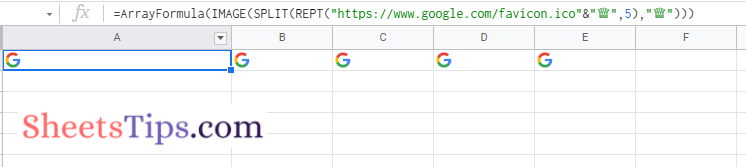### Using REPT Function to Repeat Charts In Google Sheets

We can also easily generate the bar charts within the cell in Google Spreadsheet using the REPT function. The steps to generate the bar chart in Google Sheets using the REPT function are as follow:

• 1st Step: Open the Google Spreadsheet on your device.
• 2nd Step: Now on the homepage, in column A, enter the number of times a bar chart needs to be repeated.
• 3rd Step: In column B, enter the REPT function formula (=REPT(CHAR(10074),A1)) as shown in the image below.
• 4th Step: Press the “Return” key and you will see the results as shown below.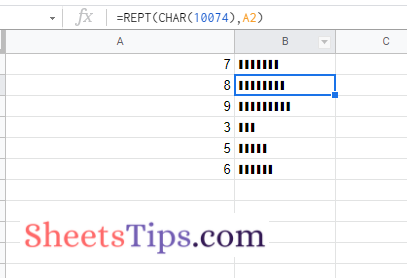### Few Other Examples of REPT Function

A few other examples using the REPT function in Google Sheets are shown below.